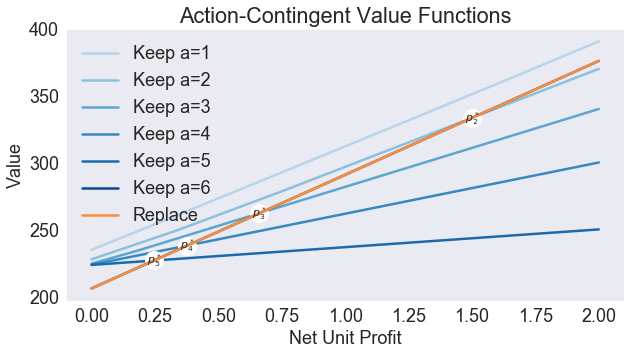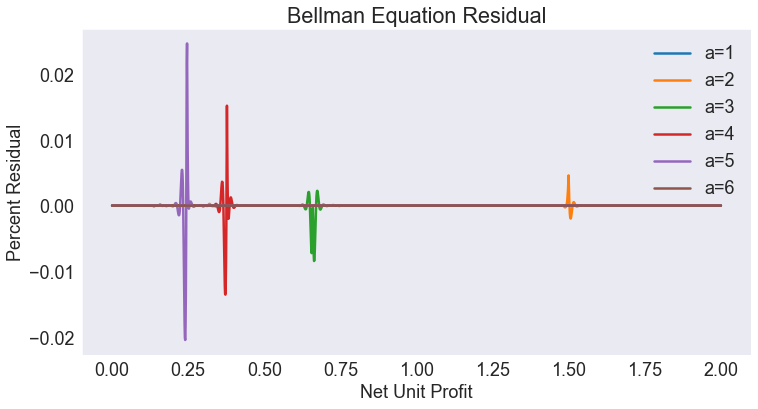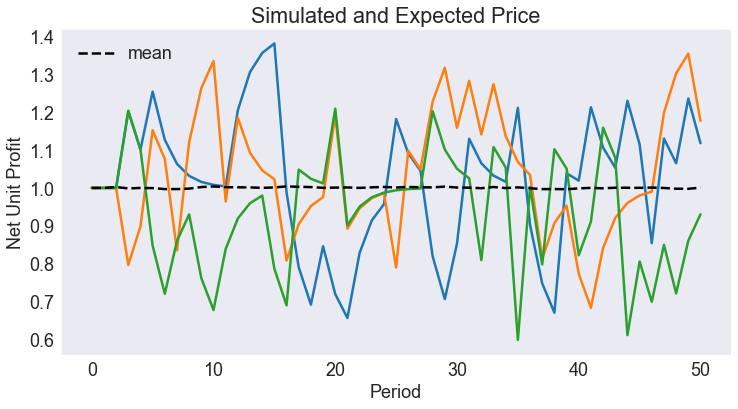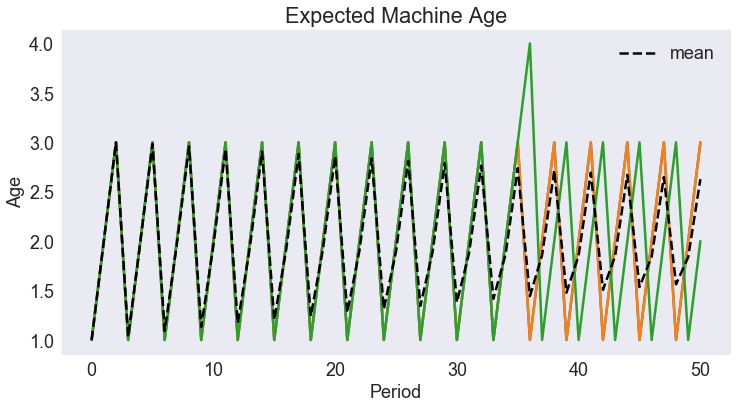# Asset Replacement Model¶

Profit-maximizing entrepreneur must decide when to replace an aging asset.

In :
import numpy as np
import matplotlib.pyplot as plt
from compecon import BasisSpline, DPmodel, qnwnorm, demo, NLP


### Model Parameters¶

The maximum age of the asset is $A=6$, production function coefficients are $\alpha =[50.0, -2.5, -2.5]$, net replacement cost is $\kappa = 40$, long-run mean unit profit is $\bar{p} = 1$, the unit profit autoregression coefficient is $\gamma = 0.5$, the standard deviation of unit profit shock is $\sigma = 0.15$, and the discount factor is $\delta = 0.9$.

In :
A       = 6
alpha   = np.array([50, -2.5, -2.5])
kappa   = 40
pbar    = 1.0
gamma   = 0.5
sigma   = 0.15
delta   = 0.9


### State space¶

The state variables are the current unit profit $p$ (continuous) and the age of asset $a\in\{1,2,\dots,A\}$.

In :
dstates = [f'a={age+1:d}' for age in range(A)]
print('Discrete states are :', dstates)

Discrete states are : ['a=1', 'a=2', 'a=3', 'a=4', 'a=5', 'a=6']


Here, we approximate the unit profit with a cubic spline basis of $n=200$ nodes, over the interval $p\in[0,2]$.

In :
n  = 200
pmin, pmax = 0.0, 2.0
basis = BasisSpline(n, pmin, pmax, labels=['unit profit'])


### Action space¶

There is only one choice variable $j$: whether to keep(0) or replace(1) the asset.

In :
options = ['keep', 'replace']


### Reward Function¶

The instant profit depends on the asset age and whether it is replaced. An asset of age $a=A$ must be replaced. If the asset is replaced, the profit is $50p$ minus the cost of replacement $\kappa$; otherwise the profit depends on the age of the asset, $(\alpha_0 + \alpha_1 a + \alpha_2 a^2)p$.

In :
def profit(p, x, i, j):
a = i + 1
if j or a == A:
return p * 50 - kappa
else:
return p * (alpha + alpha * a + alpha * a ** 2 )


### State Transition Function¶

The unit profit $p$ follows a Markov Process \begin{equation}p' = \bar{p} + \gamma(p-\bar{p}) + \epsilon \end{equation} where $\epsilon \sim N(0,\sigma^2)$.

In :
def transition(p, x, i, j, in_, e):
return pbar + gamma * (p - pbar) + e


The continuous shock must be discretized. Here we use Gauss-Legendre quadrature to obtain nodes and weights defining a discrete distribution that matches the first 10 moments of the Normal distribution (this is achieved with $m=5$ nodes and weights).

In :
m = 5
e, w = qnwnorm(m,0,sigma ** 2)


On the other hand, the age of the asset is deterministic: if it is replaced now it will be new ($a=0$) next period; otherwise its age will be $a+1$ next period if current age is $a$.

In :
h = np.zeros((2, A),int)
h[0, :-1] = np.arange(1, A)


## Model Structure¶

In :
model = DPmodel(basis, profit, transition,
i=dstates,
j=options,
discount=delta, e=e, w=w, h=h)


### SOLUTION¶

The solve method returns a pandas DataFrame.

In :
S = model.solve()

Solving infinite-horizon model collocation equation by Newton's method
iter change       time
------------------------------
0       3.7e+02    0.2599
1       4.3e+01    0.5558
2       1.2e+01    0.8437
3       5.7e-01    1.1456
4       3.7e-13    1.4555
Elapsed Time =    1.46 Seconds

Out:
unit profit i value resid j* value[keep] value[replace]
unit profit
a=1 0.000000 0.000000 0 234.982583 2.842171e-14 keep 234.982583 206.124123
0.001001 0.001001 0 235.058576 -6.159590e-07 keep 235.058576 206.209036
0.002001 0.002001 0 235.134563 -6.366804e-07 keep 235.134563 206.293949
0.003002 0.003002 0 235.210544 -2.317537e-07 keep 235.210544 206.378862
0.004002 0.004002 0 235.286520 4.292312e-07 keep 235.286520 206.463774

## Analysis¶

### Plot Action-Contingent Value Functions¶

In :
# Compute and Plot Critical Unit Profit Contributions
pcrit = [NLP(lambda s: model.Value_j(s)[i].dot([1,-1])).broyden(0.0) for i in range(A)]
vcrit = [model.Value(s)[i] for i, s in enumerate(pcrit)]

In :
fig1 = demo.figure('Action-Contingent Value Functions', 'Net Unit Profit','Value', figsize=[10,5])

cc = np.linspace(0.3,0.9,model.dims.ni)

for a, i in enumerate(dstates):
plt.plot(S.loc[i,'value[keep]'] ,color=plt.cm.Blues(cc[a]), label='Keep ' + i)
if pmin < pcrit[a] < pmax:
demo.annotate(pcrit[a], vcrit[a], f'$p^*_{a+1}$', 'wo',(0, 0), fs=11, ms=18)
print(f'Age {a+1:d}  Profit {pcrit[a]:5.2f}')

plt.plot(S.loc['a=1','value[replace]'], color=plt.cm.Oranges(0.5),label='Replace')
plt.legend()

Age 2  Profit  1.50
Age 3  Profit  0.66
Age 4  Profit  0.38
Age 5  Profit  0.25

Out:
<matplotlib.legend.Legend at 0x17bcc8cc4c8>### Plot Residual¶

In :
S['resid2'] = 100*S['resid'] / S['value']

fig2 = demo.figure('Bellman Equation Residual','Net Unit Profit','Percent Residual')
demo.qplot('unit profit','resid2','i',S)
plt.legend(model.labels.i)

Out:
<matplotlib.legend.Legend at 0x17bcc9b7e08>### SIMULATION¶

In :
T = 50
nrep = 10000
sinit = np.full(nrep, pbar)
iinit = 0
data = model.simulate(T,sinit,iinit, seed=945)
data['age'] = data.i.cat.codes + 1

In :
frm = '\t{:<10s} = {:5.2f}'

print('\nErgodic Means')
print(frm.format('Price', data['unit profit'].mean()))
print(frm.format('Age', data['age'].mean()))
print('\nErgodic Standard Deviations')
print(frm.format('Price', data['unit profit'].std()))
print(frm.format('Age', data['age'].std()))

Ergodic Means
Price      =  1.00
Age        =  2.01

Ergodic Standard Deviations
Price      =  0.17
Age        =  0.83


### Plot Simulated and Expected Continuous State Path¶

In :
data.head()

Out:
time _rep i unit profit j* age
0 0 0 a=1 1.0 keep 1
1 0 1 a=1 1.0 keep 1
2 0 2 a=1 1.0 keep 1
3 0 3 a=1 1.0 keep 1
4 0 4 a=1 1.0 keep 1
In :
subdata = data[data['_rep'].isin(range(3))]

In :
fig3 = demo.figure('Simulated and Expected Price', 'Period','Net Unit Profit')
demo.qplot('time','unit profit','_rep',subdata)
plt.plot(data[['time','unit profit']].groupby('time').mean(),'k--',label='mean')
plt.legend()

Out:
<matplotlib.legend.Legend at 0x17bccaffbc8>### Plot Expected Discrete State Path¶

In :
fig4 = demo.figure('Expected Machine Age', 'Period','Age')
demo.qplot('time','age','_rep',subdata)
plt.plot(data[['time','age']].groupby('time').mean(),'k--',label='mean')
plt.legend()

Out:
<matplotlib.legend.Legend at 0x17bccb80c88>